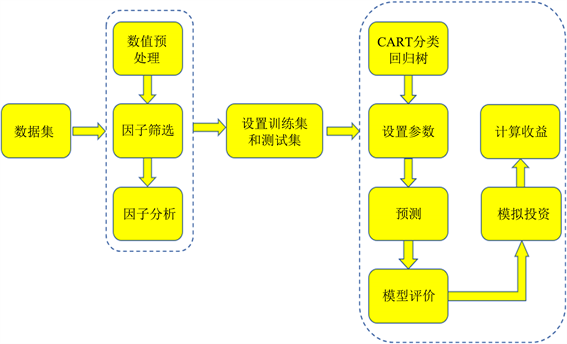﻿ 基于多因子模型和CART分类回归树的证券市场的预测及应用

# 基于多因子模型和CART分类回归树的证券市场的预测及应用Forecasting and Application for Securities Market Based on Multi-Factor Model and CART Classification Regression Tree Algorithm

Abstract: Based on Famma and French multi-factor model and CART classification regression tree algorithm, this paper forecasts the securities market. First, according to the Fama and French fmulti-factor analysis method, eight major types of company financial indicators were selected to make a multi-factor analysis. Second, the factor analysis method in statistics was adopted to extract the features of the selected factors, and five features that contribute the most weight were obtained. Then these five features and market risk indicator were taken to train a CART classification regression tree model for predicting market quarterly returns. Meanwhile 20 companies from the Shanghai Stock Exchange were randomly chosen for empirical analysis. The results show that the forecasting model has good forecasting accuracy. Especially, a simulation investment has made with history data and obtained a better performance than the average market.

1. 引言

2. 数据预处理

2.1. 数据来源

2.2. 数据处理

$\stackrel{¯}{{x}_{ij}}=\frac{{x}_{ij}-{x}_{i\mathrm{min}}}{{x}_{i\mathrm{max}}-{x}_{i\mathrm{min}}}$

2.3. 因子得分

2.3.1. 五因子得分

Fama和French五因子的因子得分计算过程如下(以市值因子SMBt为例)：

1) 按股票市值的中位数把全体股票分成小市值(S)和大市值(B)两组。

2) 按账面市值比的30%和70%分位点把样本分成高(H)、中(N)、低(L)三组，将两个指标交叉，可把全体分成SH，SN，SL，BH，BN，BL共6个组合。

3) 用同样的方法，以营运利润率和投资风格代替账面市值比，用稳健(R)、集中(N)、较弱(W)来划分盈利能力、用保守(C)、居中(N)、激进(A)来划分投资风格，可把全体分为12个组合。SR，SN，SW，BR，BN，BW和SC，SN，SA，BC，BN，BA。

4) 计算上述各组合每一期的市值加权平均季收益率，接着计算因子得分，具体公式如表2Table 2. Five-factor calculation method

2.3.2. 本文选取的31个因子得分

1) 按净资产收益率的30%和70%分位点把样本分成高(H)、中(N)、低(L)三组，可把全体公司分成H, N, L共3个组合。

2) 计算上述各组合每一期的市值加权平均季收益率，高组的市值加权平均季收益率减去低组的市值加权平均季收益率，具体公式如下：

$RO{E}_{\text{t}}=H-L$

$ELS{E}_{\text{t}}=H-L$

2.4. 筛选因子

1) 按照规模–估值筛选Table 3. The t and p values of factor regression

2) 按照规模–盈利筛选

3) 按照规模–投资筛选

2.5. 因子分析

2.6. 模型评估

$\text{MAPE}=\frac{1}{N}\underset{i=1}{\overset{N}{\sum }}|\frac{\text{real}-\text{predict}}{\text{real}}|$

$\text{RMSE}=\sqrt{\frac{1}{N}\underset{i=1}{\overset{N}{\sum }}{\left(\text{real}-\text{predict}\right)}^{2}}$

3. 模型

3.1. 多CART分类回归树

$x\in {R}_{j}⇒f\left(x\right)={\gamma }_{j}$

$T\left(x;\Theta \right)=\underset{j=1}{\overset{J}{\sum }}{\gamma }_{j}I\left(x\in {R}_{j}\right),\Theta ={\left\{{R}_{j},{\gamma }_{j}\right\}}_{1}^{J}$

$\stackrel{^}{\Theta }=\mathrm{arg}\underset{\Theta }{\mathrm{min}}\underset{j=1}{\overset{J}{\sum }}\underset{{x}_{j}\in {R}_{j}}{\sum }L\left({y}_{i},{\gamma }_{j}\right)$

$T=\frac{{T}_{1}+{T}_{2}+{T}_{3}+{T}_{4}+{T}_{5}+{T}_{6}}{6}$

3.2. 模型的实现Figure 1. Flow chart

4. 实证分析

4.1. 模型评价

4.2. 模拟投资

$CR=\underset{i=1}{\overset{n}{\prod }}\left(1+{R}_{t}\right)$

$\text{sharperatio}=\frac{E\left({R}_{p}\right)-{R}_{f}}{{\sigma }_{p}}$

20家公司四年的累计收益和夏普比率如表8Table 8. Simulation investment evaluation tableFigure 2. Investment income comparison chart

5. 结论

 Huang, C., Huang, L.-L. and Han, T.-T. (2012) Financial Time Series Forecasting Based on Wavelet Kernel Support Vector Machine. 8th International Conference on Natural Computation, Chongqing, 29-31 May 2012, 79-83.

 Yoo, P.D., Kim, M.H. and Jan, T. (2005) Machine Learning Techniques and Use of Event Information for Stock Market Prediction: A Survey and Evaluation. Computational Intelligence for Modelling, Control and Automation and International Conference on Intelligent Agents, Web Technologies and Internet Commerce, Vienna, 28-30 November 2005, 835-841.
https://doi.org/10.1109/CIMCA.2005.1631572

 Murphy, J.J. (1999) Technical Analysis of the Financial Markets. Institute of Finance, New York.

 Lee, M.-C. (2009) Using Support Vector Machine with a Hybrid Feature Selection Method to the Stock Trend Prediction. Expert Systems with Applications, 36, 10896-10904.
https://doi.org/10.1016/j.eswa.2009.02.038

 Khashei, M. and Bijari, M. (2010) An Artificial Neural Network (p, d, q) Model for Time Series Forecasting. Expert Systems with Applications, 37, 479-489.
https://doi.org/10.1016/j.eswa.2009.05.044

 Alberg, J. and Lipton, Z.C. (2017) Improving Factor-Based Quantitative Investing by Forecasting Company Fundamentals.

 Belciug, S. and Sandita, A. (2017) Business Intelligence: Statistics in Predicting Stock Market. Annals of the University of Craiova, Mathematics and Computer Science Series, 44, 292-298.
https://www.scopus.com/inward/record.uri?eid=2-s2.0-85038626695&partnerID=40&md5=35b8b79a01a6b41338d3857b3e09c36c

 Sorensen, E.H., Miller, K.L. and Ooi, C.K. (2000) The Decision Tree Approach to Stock Selection. The Journal of Portfolio Management, 27, 42-52.
https://doi.org/10.3905/jpm.2000.319781

 Andriyashin, A., HHrdle, W.K. and Timofeev, R.V. (2008) Recursive Portfolio Selection with Decision Trees. SSRN Electronic Journal.
https://doi.org/10.2139/ssrn.2894287

 Zhu, M., Philpotts, D. and Stevenson, M.J. (2012) The Benefits of Tree-Based Models for Stock Selection. Journal of Asset Management, 13, 437-448.
https://doi.org/10.1057/jam.2012.17

 Zhu, M., Philpotts, D., Sparks, R. and Stevenson, M.J. (2011) A Hybrid Approach to Combining CART and Logistic Regression for Stock Ranking. The Journal of Portfolio Management, 38, 100-109.
https://doi.org/10.3905/jpm.2011.38.1.100

 Chong, E., Han, C. and Park, F.C. (2017) Deep Learning Networks for Stock Market Analysis and Prediction: Methodology, Data Representations, and Case Studies. Expert Systems with Applications, 83, 187-205.
https://doi.org/10.1016/j.eswa.2017.04.030

 Krauss, C., Do, X.A. and Huck, N. (2017) Deep Neural Networks, Gradient-Boosted Trees, Random Forests: Statistical Arbitrage on the S&P 500. European Journal of Operational Research, 259, 689-702.
https://doi.org/10.1016/j.ejor.2016.10.031

 刘建中, 殷其威. 基于CART算法的股票价格走势预测算法研究[J]. 计算机科学与应用, 2017, 7(6): 603-614.

 Fama, E.F. and French, K.R. (2015) A Five-Factor Asset Pricing Model. Journal of Financial Economics, 116, 1-22.
https://doi.org/10.1016/j.jfineco.2014.10.010

 Fama, E.F. and French, K.R. (1993) Common Risk Factors in the Returns on Stocks and Bonds. Journal of Financial Economics, 33, 3-56.
https://doi.org/10.1016/0304-405X(93)90023-5

Top